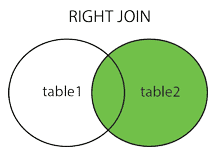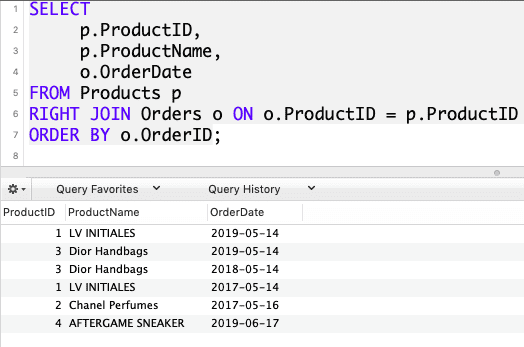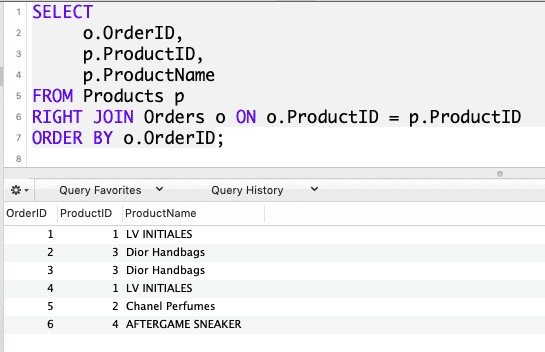# SQL Right Join: The Complete Guide

RIGHT JOIN performs a join starting with the second (right-most) table and then any matching first (left-most) table records. RIGHT JOIN and RIGHT OUTER JOIN are the same.

## SQL Right Join

SQL RIGHT JOIN returns all the records from the right table (table2) and the matched records from the left table (table1). The result is NULL from the left side when there is no match.

In some databases, the RIGHT JOIN is called RIGHT OUTER JOIN. The RIGHT JOIN clause allows us to query data from multiple tables.

See the following VENN Diagram of SQL Right Join.### Syntax

```SELECT column_name(s)
FROM table1
RIGHT JOIN table2
ON table1.column_name = table2.column_name;```

In this syntax, table1 and table2 are the left and right tables, respectively.

For each row from the table2(Right) table, the query compares it with all the rows from the table1(left) table.

If the pair of rows causes the join predicate to evaluate to true, the column values from these rows will be in the combined form to the new row, which is then included in the final result set.

If a row from the right table (table2) does not have any matching row from the table1(Left) table, the query combines column values of a row from the right table(table2) with NULL for each column value from the left table.

In short, the RIGHT JOIN clause returns all rows from the right table (table2) and matches the rows or NULL values from the left table (table1).

### Example

We need to create two tables. If you do not know how to create a SQL table, check out the SQL Create Table tutorial. Now, create the first table.

### SQL Create Tables

Run the following query to create the Products table.

``````CREATE TABLE Products (
ProductID int PRIMARY KEY AUTO_INCREMENT,
ProductName varchar(255) NOT NULL,
ProductPrice int NOT NULL
);``````

You can see the table in the database. The varchar, int is SQL Datatypes.

Now, create a second table using the following query.

``````CREATE TABLE Orders (
OrderID int PRIMARY KEY AUTO_INCREMENT,
ProductID int NOT NULL,
OrderDate date NOT NULL
);``````

So, we have created the Products and Orders table. Now, we need to fill the data into the tables.

### INSERT Data into Tables

The next thing to do is that we need to insert the data into the database.

Insert the data into the Products and Orders table using the INSERT INTO statement.

```INSERT INTO Products (`ProductName`,`ProductPrice`)
VALUES
('LV INITIALES', 60 ),
('KEEPALL BANDOULIÈRE 50', 70 ),
('KEEPALL BANDOULIÈRE 50', 80 ),
('AFTERGAME SNEAKER', 90 ),
('FRONTROW SNEAKER', 100 )```

Run the above code, and you will see the data is filled inside the Products with these values.

Now, we need to add Orders data. Let’s do that. See the below query.

```INSERT INTO Orders (`ProductID`,`OrderDate`)
VALUES
(1, NOW() ),
(3, NOW() ),
(3, NOW() ),
(1, NOW() ),
(2, NOW() ),
(4, NOW() )```

We have used the SQL NOW() function to create current data. Run the above query, and data is created inside the Orders table. So we have created two tables with the data.

Now, we will use SQL RIGHT JOIN to query the data and fetch the results.

```SELECT
p.ProductID,
p.ProductName,
o.OrderDate
FROM Products p
RIGHT JOIN Orders o ON o.ProductID = p.ProductID
ORDER BY o.OrderID;
```

See the following output.So, in the above query, we are fetching three columns.

1. p.ProductID means in the Products table fetch the ProductID.
2. p.productName means in the Products table fetch the ProductName.
3. o,orderDate means in the Orders table fetch the OrderDate.

We have Right Join Products with Orders table in which ProductID is common in both the tables.

In the Orders table, ProductID is a foreign key. Each sales order item includes one product. The link between the Products and the Orders tables is via the values in the ProductID column.

So, based on the foreign key, it maps the result in the final table.

All the right table rows are included in the final table. In our case, the right table is Orders.

So, all the Orders table rows will be added, and on the right side, if the condition is specified, then the rows will be included from the left table; otherwise, null will be returned for a particular column.

See the following query.

```SELECT
o.OrderID,
p.ProductID,
p.ProductName
FROM Products p
RIGHT JOIN Orders o ON o.ProductID = p.ProductID
ORDER BY o.OrderID;
```

See the output.So, we have seen how to create a table and then use the Right JOIN to fetch the records from two tables.

That’s it for this tutorial.

Categories SQL

This site uses Akismet to reduce spam. Learn how your comment data is processed.